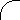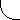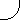"A New Redshift Interpretation"Robert V. Gentry
(arXiv:astro-ph/9806280 20 Jun 1998)

Abstract

A nonhomogeneous universe with vacuum energy, but without spacetime expansion, is utilized together with gravitational and Doppler redshifts as the basis for proposing a new interpretation of the Hubble relation and the 2.7K Cosmic Blackbody Radiation.

Hans-Dieter Radecke recently noted1 that modern cosmology's dependence on "interpretations of interpretations of observations" means that "We should not to fall victim to cosmological hubris, but stay open for any surprise." We now report what seems a major surprise—namely, the discovery of a New Redshift Interpretation (NRI) of the Hubble redshift relation and 2.7K Cosmic Blackbody Radiation (CBR) without assuming either the Friedmann-Lemaitre wavelength expansion hypothesis or the Cosmological Principle, the latter being long acknowledged as the ". . . one great uncertainty that hangs like a dark cloud over the standard model."2 Whereas the standard model and the NRI both interpret nearby galactic redshifts as Doppler shifts, they differ significantly in their interpretation of distant redshifts. This difference can be traced to two fundamentally different views of the universe. The standard model utilizes a universe governed by expanding-spacetime general relativity whereas the NRI is based on a universe governed by static-spacetime general relativity. A brief review of early twentieth-century astronomy and cosmology assists in focusing more precisely on the nature of this difference.

In 1917 Einstein applied his newly developed static-spacetime general theory of relativity to cosmology,3 and introduced a cosmological constant to maintain the universe in what was then thought to be a static condition. But Edwin Hubble's momentous 1929 discovery4 that galactic redshifts increase in proportion to their distance challenged the static universe concept. His discovery confronted cosmologists with two surprises, and they were initially unprepared to deal with either. First, they were unaware of any static-spacetime redshift interpretation which could account for increasing galactic redshifts in a real, finite-density universe. Secondly, if Hubble's results were interpreted as Doppler shifts they implied omnidirectional galactic recession, which in turn implied the existence of a universal center near the Galaxy.

In any event, whatever efforts cosmologists might have put forth to obtain a static-spacetime interpretation of Hubble's discovery were effectively cut short when their attention was soon directed to the potential cosmological implications of the hitherto virtually unnoticed results of Alexander Friedmann5 and Georges Lemaitre,6 both of whom had found expanding-spacetime solutions of the Einstein field equations in the early and mid-1920s. Their results were attractive for two reasons. First, uniform spacetime expansion showed promise for eliminating the implication of the Galaxy occupying a preferred position in the universe. Hubble spoke for most cosmologists of his time when he forthrightly admitted an extreme distaste for such a possibility, saying it should be accepted only as a last resort.7

Second, Lemaitre hypothesized that, apart from the well-known redshift due relative motion of source and observer, expanding-spacetime should cause photons everywhere to experience continuous, in-flight wavelength expansion proportional to the expansion itself.6 Thus was born the concept of spacetime expansion redshifts, given by zexp = o/e − 1; where o and e represent the magnitudes of the postulated Friedmann-Lemaitre spacetime expansion factors at observation and emission.6

Despite its critical role in standard model theory, the foregoing expression for zexp is unique in that the physical existence of has never been verified by experiment; the reason is that no method has yet been proposed to measure ; either past or present. Even so, expansion redshifts have become the cornerstone of the standard model for two reasons—namely, (1) because the experimentally determined Hubble redshift relation, z = Hr/c, can be developed as a theoretical consequence of spacetime expansion theory if the hypothesized expansion redshifts, zexp = o/e − 1, are assumed to be identical with zobs = λo/λe − 1; the observed redshifts of distant galaxies, and (2) because of their key role in providing what has previously been thought to be a unique interpretation of the 2.7K CBR. That interpretation assumes the much earlier existence of a primeval fireball radiation wherein matter/radiation decoupling occurred at about 3000K when the expansion redshift was about 1000 compared to the present. It follows that a 1000-fold redshifting of such a radiation by spacetime expansion would result in the presently observed 2.7K CBR. The Hubble relation and 2.7K CBR scenarios are widely understood as confirming the existence of expansion redshifts. Such a conclusion may be premature, however, seeing that the crucially important expansion factor, ; has yet to be experimentally verified.

This brings us to the standard model's second fundamental assumption known as the Cosmological Principle—namely, that in the large scale the universe is homogeneous and isotropic, or put in simpler terms, it is everywhere alike. This Principle was earlier noted to be the ". . . one great uncertainty that hangs like a dark cloud over the standard model."2 Uncertainty exists because, even though the Hubble relation is powerful evidence for large-scale isotropy about the Galaxy, we simply cannot confirm universal homogeneity because we lack knowing whether the Hubble relation would result if redshift measurements were made from points of observation on other galaxies.

Nevertheless the standard model requires homogeneity because in it galaxies are assumed to be comoving bodies in expanding spacetime. That is, since spacetime expansion is assumed to be uniform, comoving galactic separation must likewise be uniform, which implies that all observers, regardless of location, should see the same general picture of the universe. This is what the standard model requires, and it is observationally unprovable.

In summary, then, our mini-review of twentieth century astronomy and cosmology have revealed two reasons why we cannot be absolutely certain of Friedmann-Lemaitre expansion redshifts and the standard model's cornerstone postulate of a no-center universe governed by expanding-spacetime general relativity. First, the universal homogeneity required by the standard model is acknowledged to be observationally unprovable. Second, despite the fact that in theory all photons in the universe should be synchronously experiencing in-flight wavelength expansion in direct proportion to the instantaneous value of ; until now little attention has been given to finding a method to test this prediction. More on this later. For the present we say only that the foregoing uncertainties are sufficient to suggest the possibility that the universe may not be governed by expanding-spacetime general relativity required by the standard model. As far as is known this paper is the first attempt to seriously explore the cosmological consequences of such a possibility and, as will now be seen, the results do appear quite surprising.

The foregoing account provides the basis for understanding why the NRI attempts to account for the Hubble relation and the 2.7K CBR by using Doppler and gravitational redshifts embedded in a universe governed by static-spacetime general relativity. Without expanding spacetime there can be no Cosmological Principle, and without this Principle the Hubble relation implies the existence of a center in the NRI. In it the Hubble redshifts are now interpreted solely in terms of relativistic Doppler and Einstein gravitational redshifts, all cast within the framework of a finite, nonhomogeneous, vacuum-gravity universe with cosmic center (C) near the Galaxy.

The NRI framework assumes the widely dispersed galaxies of the visible universe are enclosed by a thin, outer shell of hot hydrogen at a distance R from the Galaxy. Thus, the volume of space enclosed by this luminous shell—assumed, for ease of calculation, to have a nearly uniform temperature of 5400K—would completely fill with blackbody cavity radiation. But the radial variation of gravitational potential within this volume means the cavity radiation temperature measured at any interior point would depend on the magnitude of the Einstein gravitational redshift between that point and the outer shell. By including relativistic vacuum energy density, ρv, and pressure, pv, into the gravitational structure of the cosmos we now show how 5400K radiation emitted at R could be gravitationally redshifted by a factor of 2000 so as to appear as 2.7K blackbody cavity radiation here at the Galaxy.

In particular, if pv is negative, then, as Novikov8 shows, ρv will be positive, and the summed vacuum pressure/energy contributions to vacuum gravity will be −2ρv. So, excluding the spherical hydrogen shell at R, the net density throughout the cosmos from C to R would be ρ − 2ρv, where ρ is the average mass/energy density. Beyond R both densities are assumed to either cancel or exponentially diminish to infinitesimal values, which effectively achieves for the NRI framework what Birkhoff's theorem did for standard cosmology. This framework is sufficient to compute the gravitational potentials needed to calculate both Hubble and 2.7K CBR redshifts in the NRI framework.

If Φ(0) and Φ(R) represent the universal potentials at C and R, then, Φ(R) = −(G/R)[M1 + MS], and Φ(0) = −G[∫0R4π(ρ − 2ρv)r · dr + MS/R], where MS is the mass of the thin, outer shell, and the net mass from C to R is M1 = 4πR3(ρ − 2ρv)/3. To find MS we employ the boundary condition Φ(0) = 0, which first yields MS = −2πR3(ρ − 2ρv) and then, by substitution, the expression Φ(R) = 2πGR2[ρ − 2ρv]/3. Explanation of Φ(0) = 0 is given in ref. (9). Gravitationally redshifting the uniform 5400K radiation at R so as to appear as the 2.7K CBR at C means that TR = 5400K and TC = 2.7K, in which case the gravitational redshift at C would be,

 z + 1 = √1 + 2Φ(0)/c² / √1 + 2Φ(R)/c² = TR / TC = 5400/2.7. (1)

If, as soon to be explained, we let ρv = 8.790 × 10−30 g/cm3 and ρ = 2 × 10−31 g/cm3, then Eq. (1) yields R 1.362 × 1028 cm, or about 14.24 × 109 ly. These are the conditions which allow the 2.7K CBR to be interpreted as blackbody cavity radiation. Now consider the Hubble relation.

Interpreting the Hubble relation in the NRI framework assumes that distant galaxies may have both radial and transverse velocity components relative to C. Thus the appropriate redshift equation is given by the standard expression,10

 z = [1 − v→ · k^/c]√1 + 2Φ(0)/c² / √1 + 2Φ(r)/c² − v²/c² − 1 (2)

which describes how light emitted from a distant galaxy moving at velocity v—with the unit vector k^ pointing from source to observer—will be redshifted by a combination of gravitational and special relativistic Doppler effects. In Eq. (2) r is the distance from C to an arbitrary galaxy where the gravitational potential is,

 Φ(r) = −G[(r−1 ∫0r 4π(ρ − 2ρv)r2 · dr) + ∫rR 4π(ρ − 2ρv)r · dr + MS/R] (3)

Substituting the expression MS = −2πR3(ρ − 2ρv), we arrive at 2Φ(r)/c2 = 4πGr2[ρ − 2ρv]/3c2, which together with the radial velocity, vr = −v · k^, and boundary condition Φ(0) = 0, allows Eq. (2) to be rewritten as,

 z = [1 + vr/c] / √1 + 4πGr²(ρ − 2ρv)/3c² − v²/c² − 1. (4)

To obtain the Hubble relation from Eq. (4) we note that if 2ρv > ρ, then the (ρ − 2ρv) density factor will cause any galaxy located at a distance r from C to experience an outward radial acceleration, r¨ = −GM/r2, due to the enclosed negative mass M = 4πr3(ρ − 2ρv)/3. This leads to the equation r¨ = br, where b = 4πG(2ρvρ)/3. Its solution is r = rgexp√bt, where rg is a galaxy-specific, initial condition parameter. Taking its proper time derivative leads to the expression vr = r = √br, which is of course the Hubble velocity-distance relation, vr = Hr, with H = √b.

If ρ = 2 × 10−31g-cm−3 and H = 68 km-s−1-Mpc−1 = 2.203 × 10−18s−1 are substituted into H2 = b = 4πG(2ρvρ)/3, then the vacuum density is ρv 8.79 × 10−30g-cm−3. This value for H was earlier utilized to calculate R 14.24 billion ly. And substitution of this value of the vacuum density in ρvc2 = Gmv6c4/4, as given by Novikov8, yields the average mass, mv, of the virtual particles emitted by the vacuum. If me is the electron mass, then mv 82me for ρ = 2 × 10−31g-cm−3.

If we consider the case for dark matter, where ρ = 2 × 10−30g-cm−3, then ρv 9.79 × 10−30g-cm−3 and mv 83me. Thus the use of dark matter density in the NRI produces only negligible changes in mv and ρv, which has the important consequence of justifying the use of ρv as an adjustable parameter in the expression H2 = 4πG(2ρvρ)/3. Indeed, because the right side of this expression occurs explicitly in the NRI redshift Equation (4), it's possible to restate it in terms of H2 without a density term. (See further discussion in the next paragraph.) This means the results already obtained, as well as those that now follow, apply equally to a universe with or without the assumption of dark matter.

Further interpretation of Eq. (4) requires evaluating the v2/c2 = (vr2 + vθ2)/c2 term. The most general case assumes a possible transverse velocity component such that vθ = ugvr —where ug is an undetermined, galactic-specific parameter constrained only by the v < c condition— thus leading to v2/c2 = (1 + ug2)vr2/c2 = (1 + ug2)(Hr/c)2. Thus, Eq. (4) can be rewritten as,

 z = (1 + Hr/c) / √1 − (2 + ug²)(Hr/c)² − 1. (5)

For small r, Eq. (5) reduces to the Hubble redshift relation, z = Hr/c, which means the NRI framework successfully interprets both it and the 2.7K CBR. Even the latter's microtemperature variations11 can be interpreted as temperature variations in the 5400K outer shell. Going further, the equation, r¨ = br, with b as given above, essentially duplicates12 the inflationary expansion relation, ¨ = {8πGρf / 3}.

The NRI framework also succeeds in interpreting the observed variation of CBR temperature with redshift. When Songaila et al.13 investigated this topic, their measurement yielded a CBR temperature of 7.4 ± 0.8 K for z = 1.776. The NRI framework's prediction is obtained by substituting r for R in Eq. (1), from which it follows that the CBR temperature should vary spatially with r and z as Tz = TC / 1 + 2Φ(r)/c² = (z + 1)TC. For TC = 2.726K, then T1.776 = 7.57K, which agrees with experiment and duplicates the standard cosmology's prediction, but without employing the expansion hypothesis, inflationary or otherwise.

Can the NRI framework also be applied to the interpretation of quasar redshifts? Perhaps so if we focus on the recent confirmation of the paucity of quasars with z > 4, and their near absence for z > 5.14 If, for example, we assume ug2 0.5 and vθ = 0.7c might apply to some of the most distant quasars, then Eq. (5) reveals that z increases from 4.3 at 8.8 × 109 ly to 534 at 9.1263 × 109 ly. Could such a rapid increase in z be interpreted as one reason for the sparsity of very high redshift quasars? If so, quasars with z > 4.3 might have proper motions of ~ 15 μas/y.

Next, consider Hubble Deep Field. It is of interest to note that its analysis shows that angular diameters of the most distant galaxies do not go through a minimum and then increase as predicted by the standard cosmology.15 Instead angular diameters continue to diminish. Standard cosmology's prediction is of course traceable to its underlying assumption of spacetime expansion. Since the NRI framework does not have that assumption, it can quite naturally interpret Hubble Deep Field results as evidence of the applicability of the general astronomical rule-of-thumb for galaxies—the further the distance, the smaller the angular diameter.

The NRI framework's apparent ability to interpret a variety of astronomical and astrophysical observations with Einstein's gravitational and Doppler redshifts, without the addition of the Friedmann-Lemaitre spacetime wavelength expansion hypothesis and the Cosmological Principle, was such a surprise that it seemed quite natural to extend this study and reanalyze the physics underlying the expansion hypothesis itself.

Our ongoing investigations on this topic have led us to uncover an intriguing, new test of the expansion hypothesis.16 The end result is yet another surprise almost on par with finding that the NRI framework is an alternate interpretation of the Hubble relation and the 2.7K CBR.

References

1. Hans-Dieter Radecke, Science 275, 603 (1997).

2. S. Weinberg, The First Three Minutes, Bantam Books 1977, p. 111.

3. A. Einstein, Preuss. Akad. Wiss. Berlin, Sitsber. 142 (1917). English reprint in The Principle of Relativity, Dover Publications, pp. 177-198.

4. E. P. Hubble, Proc. Nat. Acad. Sci. 15, 168 (1929).

5. A. Friedmann, Z. Phys. 10, 377 (1922). English reprint in A Sourcebook in Astronomy and Astrophysics, Eds. K. R. Lang and Owen Gingerich, Harvard University Press 1979, pp. 838-843.

6. G. Lemaitre, Annales Societe Scientifique Bruxelles A47, 49 (1927). English reprint in A Sourcebook in Astronomy and Astrophysics, Eds. K. R. Lang and O. Gingerich, Harvard University Press 1979, pp. 844-848.

7. E. P. Hubble, The Observational Approach to Cosmology, The Clarendon Press 1937, pp. 50-51.

8. I. D. Novikov, Evolution of the Universe, Cambridge University Press 1983, pp. 52-63.

9. Gravity in the NRI is the sum of local and universal potentials. Thus two clocks at distances s1 and s2 from Earth's center should run at rates that differ by 1 + 2[Φ(0) + φ(s1)]/c² / 1 + 2[Φ(0) + φ(s2)]/c², where φ(s) is the Earth's local potential. The synchronization of GPS atomic clocks with the 1 + 2φ(s1)/c² / 1 + 2φ(s2)/c² ratio is consistent with Φ(0) = 0.

10. M. D. Harkins, Radio Science, 14, 671 (1979).

11. G. F. Smoot et al. Ap. J. 396, L1-L5 (1992).

12. A. H. Guth, in Observational tests of Cosmological Inflation, Eds. T. Shanks et al. (Kluwer Academic Publishers 1991) pp. 1-21.

13. A. Songaila. et al., Nature 371, 43 (1994).

14. P. A. Shaver et al., Nature 384, 439 (1996).

15. H. C. Ferguson, R. L. Williams, and L.L. Cowie, Physics Today V.50, No. 4, 24 (1997).

16. R. V. Gentry, see accompanying preprint gr-gc/9806061.